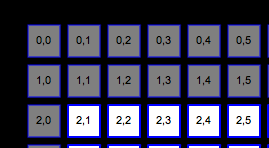# Spiraling through a matrix

As I was saying in my previous post, I ran into a little trickiness with circling through the squares in my matrix of squares. Now there may be a better way to do this, and by all means if there is let me know, but here is the best way I figured out how to do it.

```
var TOTAL_ROWS:int = 16;

var TOTAL_COLS:int = 16;

var vectorArr:Array = new Array();

for (var r:int = 0; r= leftCol; lowerCols--)//going left on lower part
{
trace('LEFT FOR '+vectorArr[downRows-1][lowerCols].id);
}

planeRowLength--;
for(var upRows:int = planeRowLength; upRows > rows+1; upRows--)//going back up left side
{
trace('UP FOR '+vectorArr[upRows-1][leftCol].id);
}
leftCol++;
}

```

In this code we create a objects inside group of arrays inside an array for rows and columns and the object is just there for testing purposes so we can see where we are when passing through this.

We first pass through the first row iterating along the row through each column.

```for(var cols:int = leftCol; cols
Once we've reached the end of that we progress down the last available column. Following this for loop we decrement the column length so when we come back to it we'll be traveling down one column to the left of this one, working our way inward.
for(var downRows:int = rows+1; downRows
Now, we'll move to the left towards column 0, after which we will again decrement a variable but this time the rows length so we can work our way inwards this way as well.
for(var lowerCols:int = planeColLength-1; lowerCols >= leftCol; lowerCols--)//going left on lower part
{
trace('LEFT FOR '+vectorArr[downRows-1][lowerCols].id);
}

planeRowLength--;

Finally we work our way back up to the point almost where we started from only one higher in the rows number than the current row is.
And since the rows are incrementing and the planeRowLength is decrementing we work our way inward until we're out of numbers.
for(var upRows:int = planeRowLength; upRows > rows+1; upRows--)//going back up left side
{
trace('UP FOR '+vectorArr[upRows-1][leftCol].id);
}
leftCol++;

For a more visual idea of what it's doing click on the image below. If you would like the FLA for the below swf there's a link in the swf to download it.You'll need TweenMax if you wish to run the fla. Once you have that, set your library class path to the appropriate location.
```